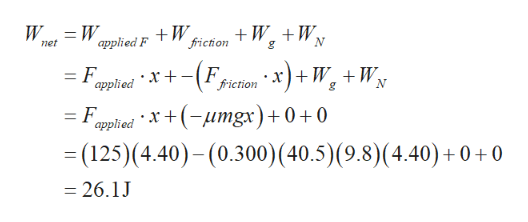# A 40.5 kg box initially at rest is pushed 4.40 m along a rough, horizontal floor with a constant applied horizontal force of 125 N. If the coefficient of friction between box and floor is 0.300, find the following.(d) the work done by the gravitational force J(e) the change in kinetic energy of the box J(f) the final speed of the box m/s

Question
2 views

A 40.5 kg box initially at rest is pushed 4.40 m along a rough, horizontal floor with a constant applied horizontal force of 125 N. If the coefficient of friction between box and floor is 0.300, find the following.

(d) the work done by the gravitational force
J

(e) the change in kinetic energy of the box
J

(f) the final speed of the box
m/s

check_circle

Step 1

(d). the work done by the gravitational force is zero. Because the gravitational force on the box and displacement of the box are perpendicular. So the gravitational force on the box is pointed downward.

Step 2

(e).
The net work done is equal to the sum of work done by the applied force, work done by the frictional force, work done by the normal force, and work done by the gravitational force.help_outlineImage TranscriptioncloseW friction W W applied F et = F. applied x+Ffiction * x)+ W. + W, = F x+(-umgx)+ 0 +0 applied =(125)(4.40)-(0.300) (40.5) (9.8) (4.40) +0 +0 =26.1J fullscreen
Step 3

According to the work energy theorem, the net work done is...

### Want to see the full answer?

See Solution

#### Want to see this answer and more?

Solutions are written by subject experts who are available 24/7. Questions are typically answered within 1 hour.*

See Solution
*Response times may vary by subject and question.
Tagged in

### Work,Power and Energy